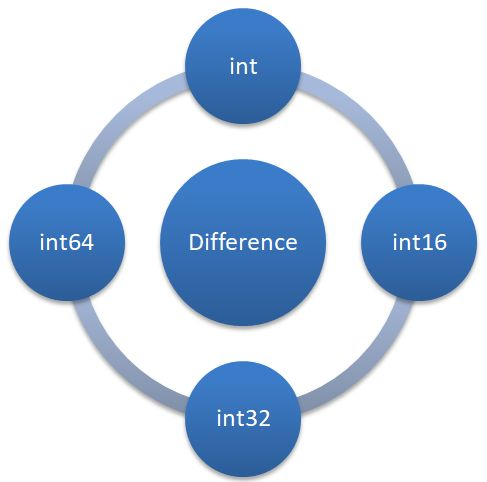﻿ C# Difference Between int, int16, int32 and int64 - TutorialsTrend
This Angular tutorial helps you get started with Angular quickly and effectively through many practical examples.
`

# C# - Difference Between int, int16, int32 and int64

In C#, int, Int32, and Int64 are all numeric data types that can hold integer values, but they differ in their range and memory usage. This cause bugs and performance issues into the code. In this article, I would like to explain the different behavior of integer type.#### What is FCL?

FCL stands for Framework Class Library. It is a collection of pre-built classes and data types that are provided by the .NET Framework. The FCL includes a variety of data types that are used to represent different types of data, such as integers, strings, dates, times, and more.

#### Diffrence Between int, int16, int32 and int64 DataTypes

The below points defines the diffrence between int, int16, int32 and int64 datatypes.

#### 1. int

1. It is a primitive data type
2. int is a 32-bit signed integer data type
3. It can hold values between approximately -2147483648 and maximum +2147483647.

#### 2. Int16

1. It is a FCL type.
2. It is a 16-bit signed integer data type.
3. It can hold values between approximately minimum -32768 and maximum +32767 capacity.

#### 3. Int32

1. It is a FCL type.
2. It is a 32-bit signed integer data type.
3. It can hold values between approximately minimum -2147483648 and maximum +2147483647.
4. It is just an alias for int.

#### 4. Int64

1. It is a FCL type.
2. It is a 64-bit signed integer data type.
3. It can hold values between approximately minimum –9,223,372,036,854,775,808 and maximum 9,223,372,036,854,775,807.
4. It takes up twice as much memory as int or Int32

Example

``````int checkInt = 20; // This is a 32-bit integer
Int16 checkInt16 = 30; // This is a 16-bit integer
Int32 checkInt32 = 40; // This is the same as 'int'
Int64 checkInt64 = 50; // This is a 64-bit integer

// Add the values together and store in a new variable
Int64 result = checkInt + checkInt16 + checkInt32 + checkInt64;

// Display the result
Console.WriteLine("Result: " + result);``````

Prev Next